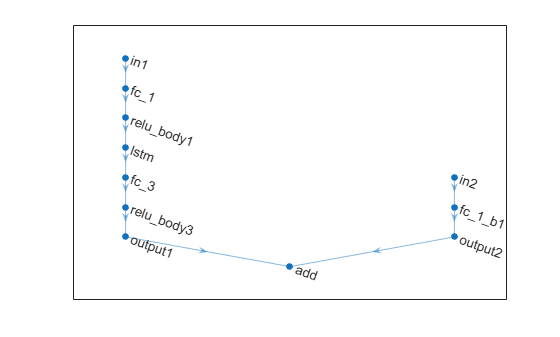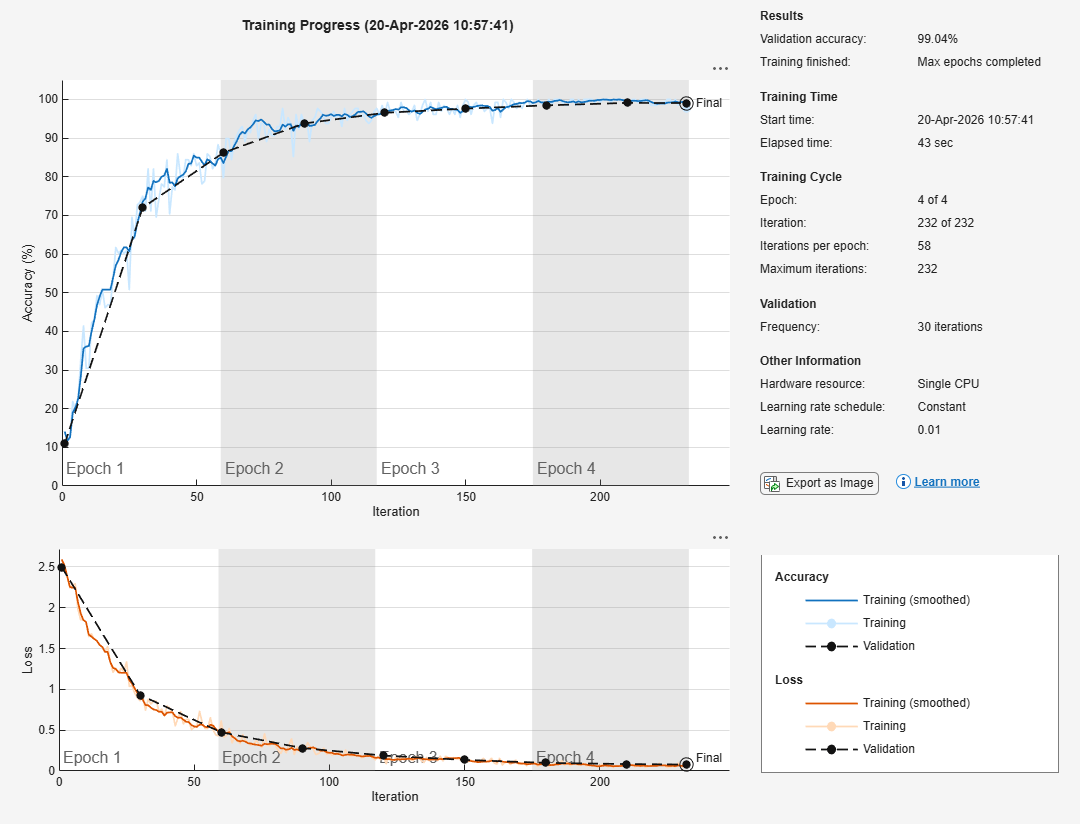## Analyze Network for Code Generation

You can analyze code generation compatibility of deep learning networks by using the `analyzeNetworkForCodegen` function. Use the network code generation analyzer to validate a `SeriesNetwork`, `DAGNetwork`, and `dlnetwork` for non-library and library targets and detect problems before code generation. Supported library targets include `MKL-DNN`, `ARM Compute`, `CMSIS-NN`, `ARM Compute Mali`, `cuDNN`, and `TensorRT`. Problems that `analyzeNetworkForCodegen` detects include unsupported layers for code generation, network issues, built-in layer specific issues, and issues with custom layers.

The `analyzeNetworkForCodegen` function requires the MATLAB® Coder™ Interface for Deep Learning and GPU Coder™ Interface for Deep Learning support packages. To download and install support package, use the Add-On Explorer. You can also download the support packages from MathWorks GPU Coder Team and MathWorks MATLAB Coder Team.

### Check `dlnetwork` for Code Generation Compatibility

This example shows how to check code generation compatibility of multi-input `dlnetwork` by using the `analyzeNetworkForCodegen` function.

The example checks code generation support for the following targets:

• Library free code generation.

• ARM® Compute Library.

• ARM Compute Library for Mali GPU.

• Intel® Math Kernel Library for Deep Neural Networks (Intel MKL-DNN).

• Common Microcontroller Software Interface Standard - Neural Network (CMSIS-NN) library.

• NVidia® CUDA® Deep Neural Network library (cuDNN).

• NVIDIA TensorRT high performance deep learning inference optimizer and run-time library.

Define Network Architecture

Construct a network with two branches. The network takes two inputs, with one input per branch. Connect the branches using an addition layer.

```layersBranch1 = [ sequenceInputLayer(1,"Name","in1","Normalization","none") fullyConnectedLayer(32,"Name","fc_1") reluLayer("Name","relu_body1") lstmLayer(32,"Name","lstm", "OutputMode","last") fullyConnectedLayer(32,"Name","fc_3") reluLayer("Name","relu_body3") fullyConnectedLayer(4,"Name","output1") additionLayer(2,"Name","add")]; layersBranch2 = [ imageInputLayer([5 5 3],"Name","in2","Normalization","none") fullyConnectedLayer(4,"Name","fc_1_b1") reluLayer("Name","output2")]; lgraph = layerGraph(layersBranch1); lgraph = addLayers(lgraph, layersBranch2); lgraph = lgraph.connectLayers('output2', 'add/in2'); figure plot(lgraph)```Create the dlnetwork.

`dlnet = dlnetwork(lgraph);`

Analyze Network for Code Generation

Run the `analyzeNetworkForCodegen` function for `mobilenetv2`, specifying the target libraries to analyze. The `analyzeNetworkForCodegen` function requires the MATLAB® Coder™ Interface for Deep Learning and the GPU Coder™ Interface for Deep Learning support packages. To install the required support packages, use the Add-On Explorer.

```targetLibraries = {'none','arm-compute','arm-compute-mali',... 'mkldnn','cmsis-nn','cudnn', 'tensorrt'}; S = analyzeNetworkForCodegen(dlnet,TargetLibrary = targetLibraries);```
``` Supported NetworkDiagnostics LayerDiagnostics _________ ___________________________________________________________________________________________________________________________________________________________ _________________________________________________________________________________________________________________________________________________________________________________ none "Yes" "" "" arm-compute "Yes" "" "" arm-compute-mali "No" "Found 1 issue(s). View network diagnostics." "Found 2 unsupported layer type(s). View incompatible layer types." mkldnn "Yes" "" "" cmsis-nn "No" "Found 1 issue(s). View network diagnostics." "Found 2 unsupported layer type(s). View incompatible layer types." cudnn "Yes" "" "" tensorrt "Yes" "" "" ```

Display the layer diagnostics for CMSIS-NN code generation.

`S(5).LayerDiagnostics`
```ans=4×3 table LayerName LayerType Diagnostics ____________ _______________ _________________________ "add" "AdditionLayer" "Unsupported layer type." "relu_body1" "ReLULayer" "Unsupported layer type." "relu_body3" "ReLULayer" "Unsupported layer type." "output2" "ReLULayer" "Unsupported layer type." ```

Display the network diagnostics for CMSIS-NN code generation.

`S(5).NetworkDiagnostics.Diagnostics`
```ans = "Code generation for cmsis-nn library does not support dlnetwork objects with combinations of sequence and non-sequence input layers. " ```

### Analyze Classification Network for Code Generation Compatibility

This example shows how to create a simple convolutional neural network for deep learning classification and test the network for code generation compatibility. The example demonstrates how to:

• Load and explore image data.

• Define the network architecture.

• Specify training options and train the network.

• Predict the labels of new data and calculate the classification accuracy.

• Analyze the deep learning network for code generation and report network and layer compatibility issues by using `analyzeNetworkForCodegen`.

The `analyzeNetworkForCodegen` function requires the MATLAB® Coder™ Interface for Deep Learning and the GPU Coder™ Interface for Deep Learning support packages. To install the required support packages, use the Add-On Explorer.

Load the digit sample data as an image datastore. Display some of the images in the datastore.

```digitDatasetPath = fullfile(matlabroot,'toolbox','nnet','nndemos', ... 'nndatasets','DigitDataset'); imds = imageDatastore(digitDatasetPath, ... 'IncludeSubfolders',true,'LabelSource','foldernames'); figure; perm = randperm(10000,20); for i = 1:20 subplot(4,5,i); imshow(imds.Files{perm(i)}); end```Check the size of the first image in `digitData`. Each image is 28-by-28-by-1 pixels.

```img = readimage(imds,1); size(img)```
```ans = 1×2 28 28 ```

Divide the data into training and validation data sets, so that each category in the training set contains 750 images, and the validation set contains the remaining images from each label.

```numTrainFiles = 750; [imdsTrain,imdsValidation] = splitEachLabel(imds,numTrainFiles,'randomize');```

Define Network Architecture

Define the convolutional neural network architecture.

```layers = [ imageInputLayer([28 28 1]) convolution2dLayer(3,8,'PaddingValue',5,'Name','conv1') batchNormalizationLayer reluLayer maxPooling2dLayer(2,'Stride',2) convolution2dLayer(3,16,'Padding','same','Name','conv2') batchNormalizationLayer reluLayer maxPooling2dLayer(2,'Stride',2) convolution2dLayer(3,32,'Padding','same','Name','conv3') batchNormalizationLayer reluLayer fullyConnectedLayer(10) softmaxLayer classificationLayer];```

Specify Training Options and Train Network

Train the network using stochastic gradient descent with momentum (SGDM) with an initial learning rate of 0.01. Set the maximum number of epochs to 4. Monitor the network accuracy during training by specifying validation data and validation frequency. Shuffle the data every epoch. Turn on the training progress plot, and turn off the command window output.

```options = trainingOptions('sgdm', ... 'InitialLearnRate',0.01, ... 'MaxEpochs',4, ... 'Shuffle','every-epoch', ... 'ValidationData',imdsValidation, ... 'ValidationFrequency',30, ... 'Verbose',false, ... 'Plots','training-progress');```

Train the network using the architecture defined by `layers`, the training data, and the training options. The training progress plot shows the mini-batch loss and accuracy and the validation loss and accuracy.

`net = trainNetwork(imdsTrain,layers,options);`Classify Validation Images and Compute Accuracy

Predict the labels of the validation data using the trained network, and calculate the final validation accuracy. Accuracy is the fraction of labels that the network predicts correctly. In this case, more than 99% of the predicted labels match the true labels of the validation set.

```YPred = classify(net,imdsValidation); YValidation = imdsValidation.Labels; accuracy = sum(YPred == YValidation)/numel(YValidation)```
```accuracy = 0.9908 ```

Analyze Network for Code Generation

To check the network for code generation compatibility, run `analyzeNetworkForCodegen`. By default, the function validates against a set of default CPU and GPU deep learning library targets. `analyzeNetworkForCodegen` returns a 1-by-N structure containing the analysis results.

`S = analyzeNetworkForCodegen(net)`
``` Supported LayerDiagnostics _________ _______________________________________________________________________________________________________________________________________________________________________ none "No" "Found 1 issue(s) in 1 layer(s). View layer diagnostics." arm-compute "No" "Found 1 issue(s) in 1 layer(s). View layer diagnostics." mkldnn "No" "Found 1 issue(s) in 1 layer(s). View layer diagnostics." cudnn "No" "Found 1 issue(s) in 1 layer(s). View layer diagnostics." tensorrt "No" "Found 1 issue(s) in 1 layer(s). View layer diagnostics." ```
```S=1×5 struct array with fields: TargetLibrary Supported NetworkDiagnostics LayerDiagnostics IncompatibleLayerTypes ```

To view the layer issues in the network for the cuDNN target, use the following command. Alternatively, you can click on the `View layer diagnostics` hyperlink to display the layer issues.

`S(4).LayerDiagnostics`
```ans=1×3 table LayerName LayerType Diagnostics _________ ____________________ _______________________________________________________________________________________________________________________________________________ "conv1" "Convolution2DLayer" "Layer 'conv1' has a non-default padding value. Code generation for nnet.cnn.layer.Convolution2DLayer only supports padding value equal to 0. " ```

The first `convolution2dLayer` (`conv1`) has non-zero padding value. For code generation, the `PaddingValue` parameter must be equal to 0, which is the default value.

Fix Network Issues and Retrain

In this example, the padding value of the convolution layer can be set to zero.

`layers(2) = convolution2dLayer(3,8,'PaddingValue',0,'Name','conv1');`

Retrain the modified network using the following training options.

```options = trainingOptions('sgdm', ... 'InitialLearnRate',0.01, ... 'MaxEpochs',4, ... 'Shuffle','every-epoch', ... 'ValidationData',imdsValidation, ... 'ValidationFrequency',30, ... 'Verbose',false, ... 'Plots','none'); net = trainNetwork(imdsTrain,layers,options);```

Check the modified network for code generation compatibility.

`S = analyzeNetworkForCodegen(net)`
``` Supported _________ none "Yes" arm-compute "Yes" mkldnn "Yes" cudnn "Yes" tensorrt "Yes" ```
```S=1×5 struct array with fields: TargetLibrary Supported NetworkDiagnostics LayerDiagnostics IncompatibleLayerTypes ```

The `analyzeNetworkForCodegen` function reports no issues. The network is now ready for code generation.